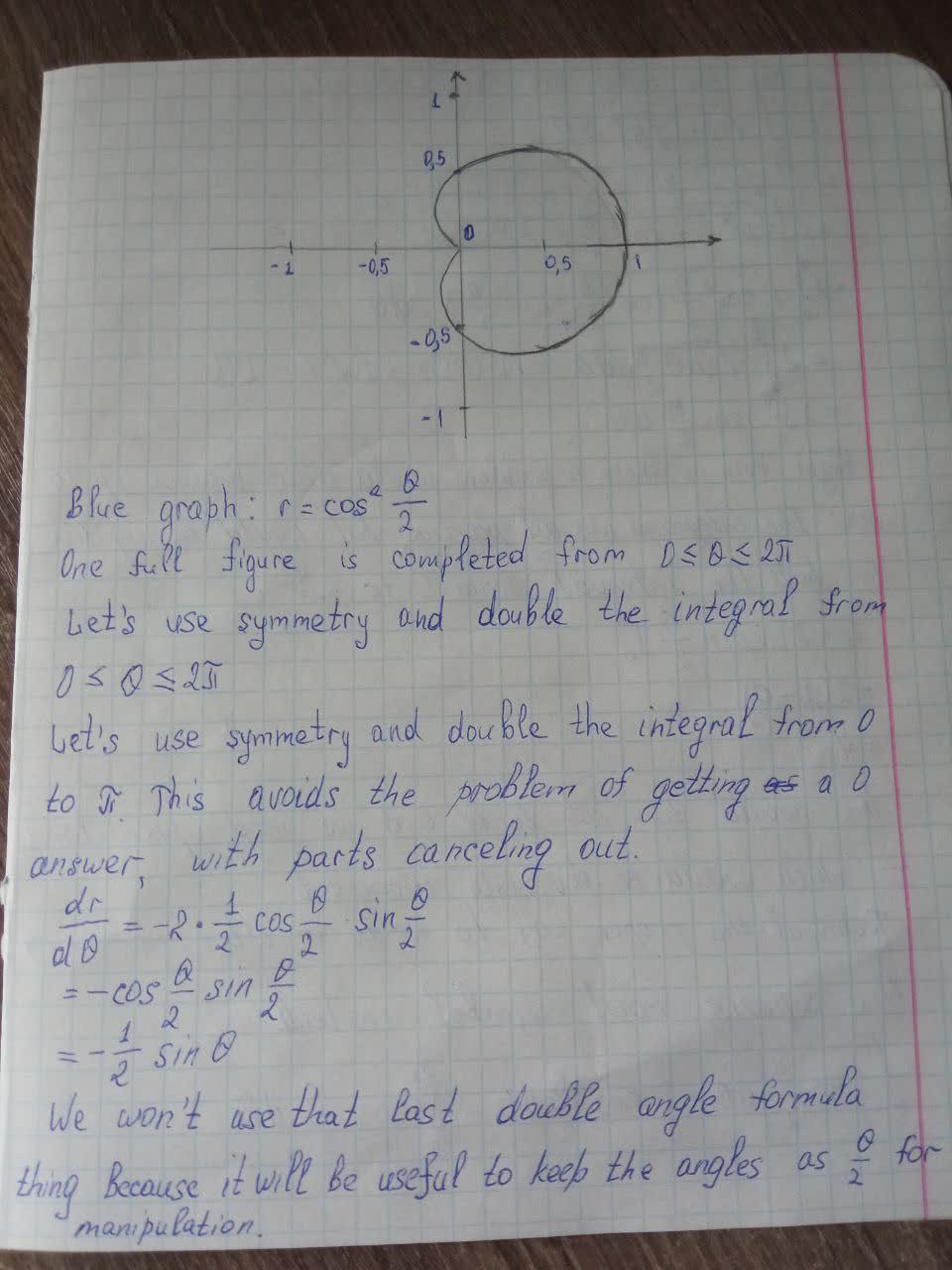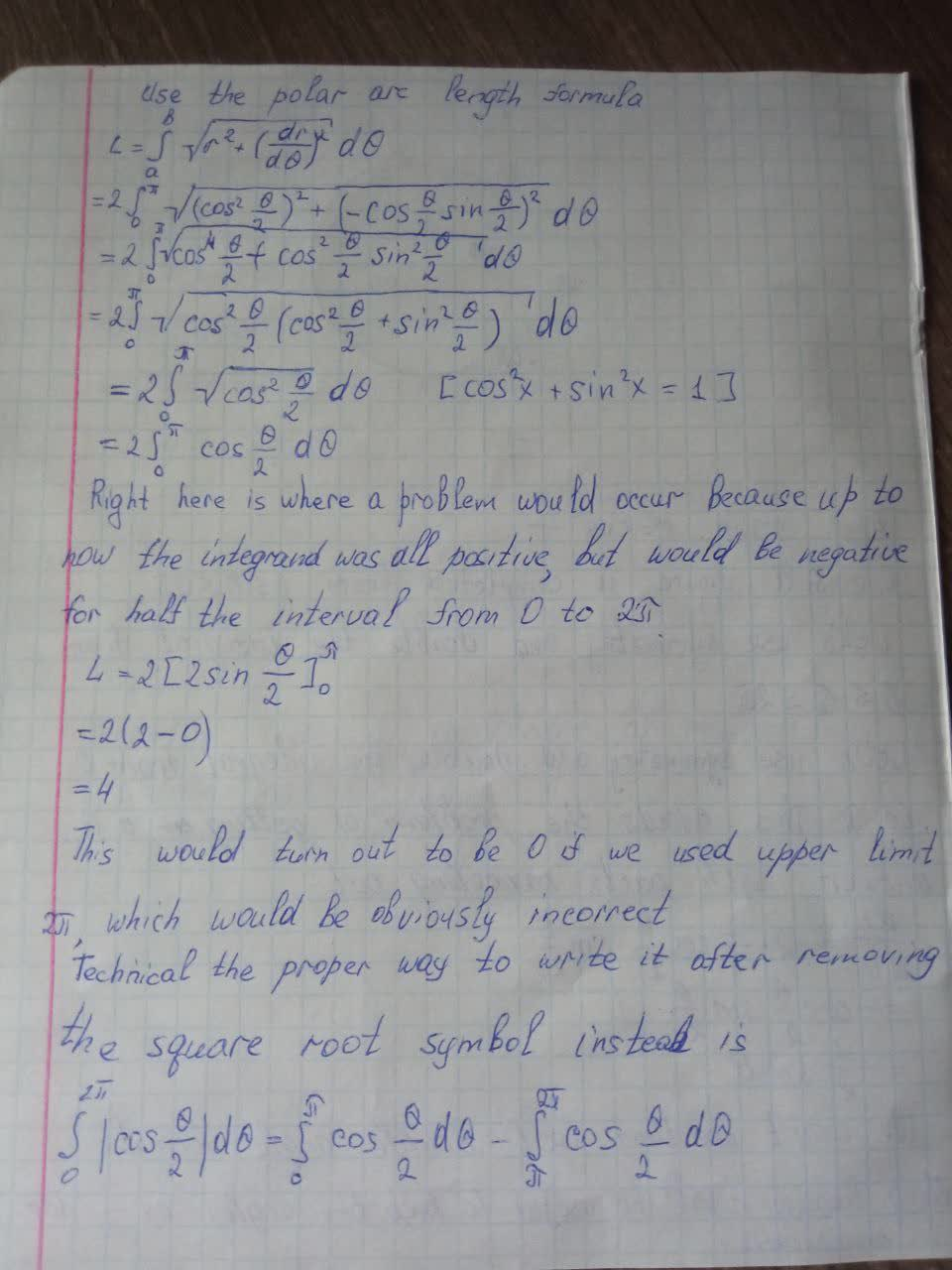Question# Find the exact length of the curve. Use a graph to determine the parameter interval.r=\cos^2(\theta/2)

Parametric equations
ANSWEREDFind the exact length of the curve. Use a graph to determine the parameter interval.
$$r=\cos^2(\frac{\theta}{2})$$